Subsections

# Langevin Equation

## Problem

We have one particle surrounded by small molecules. At t=0 it had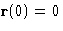. We want to calculate its position at the time t.

Assumptions:

• The velocity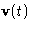is the slowest mode
• Friction coefficient is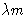## Equation

By definition,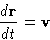(1)
We already obtained equation for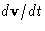: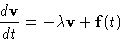(2)
with random force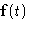: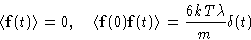(3)

This is called Langevin equation. Langevin equation describes system with white noise acting on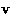.

## Solution

Let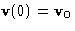. In equilibrium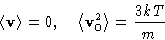(4)

Solution of Langevin equation foris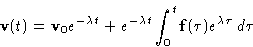(5)

Solution for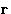is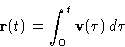1.
Integration of the first term in (5):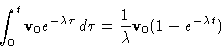2.
Second term--by parts: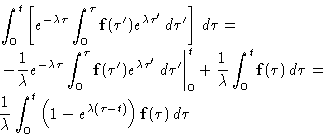We obtained: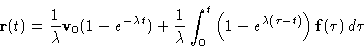Averages: we know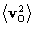and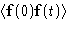. The cross-average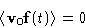1.
First term gives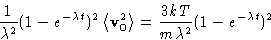2.
Second term gives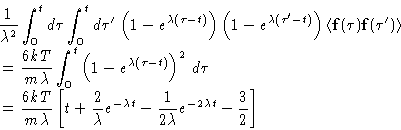## Interpretation

We obtained: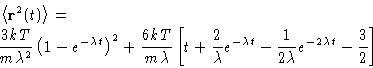(6)
At small timesAt large t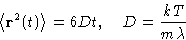The large time limit could be obtained from the Wiener equation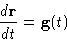(7)
with white noise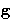: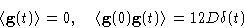In the limit of small t Wiener equation does not work!

White noise in Wiener equation is the consequence of the averaging out a fast process--in our case, velocity relaxation!Next: Diffusion Approach. Focker-Planck Equation Up: Brownian Motion and Focker-Planck Previous: Brownian Motion and Focker-Planck

© 1997 Boris Veytsman and Michael Kotelyanskii
Sun Nov 2 18:50:28 EST 1997